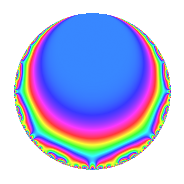# Properties

 Label 84.1.pLevel 84 Weight 1 Character orbit p Rep. character $$\chi_{84}(53,\cdot)$$ Character field $$\Q(\zeta_{6})$$ Dimension 2 Newforms 1 Sturm bound 16 Trace bound 0

# Related objects

## Defining parameters

 Level: $$N$$ = $$84 = 2^{2} \cdot 3 \cdot 7$$ Weight: $$k$$ = $$1$$ Character orbit: $$[\chi]$$ = 84.p (of order $$6$$ and degree $$2$$) Character conductor: $$\operatorname{cond}(\chi)$$ = $$21$$ Character field: $$\Q(\zeta_{6})$$ Newforms: $$1$$ Sturm bound: $$16$$ Trace bound: $$0$$

## Dimensions

The following table gives the dimensions of various subspaces of $$M_{1}(84, [\chi])$$.

Total New Old
Modular forms 14 2 12
Cusp forms 2 2 0
Eisenstein series 12 0 12

The following table gives the dimensions of subspaces with specified projective image type.

$$D_n$$ $$A_4$$ $$S_4$$ $$A_5$$
Dimension 2 0 0 0

## Trace form

 $$2q$$ $$\mathstrut -\mathstrut q^{3}$$ $$\mathstrut -\mathstrut q^{7}$$ $$\mathstrut -\mathstrut q^{9}$$ $$\mathstrut +\mathstrut O(q^{10})$$ $$2q$$ $$\mathstrut -\mathstrut q^{3}$$ $$\mathstrut -\mathstrut q^{7}$$ $$\mathstrut -\mathstrut q^{9}$$ $$\mathstrut -\mathstrut 2q^{13}$$ $$\mathstrut +\mathstrut q^{19}$$ $$\mathstrut +\mathstrut 2q^{21}$$ $$\mathstrut -\mathstrut q^{25}$$ $$\mathstrut +\mathstrut 2q^{27}$$ $$\mathstrut +\mathstrut q^{31}$$ $$\mathstrut +\mathstrut q^{37}$$ $$\mathstrut +\mathstrut q^{39}$$ $$\mathstrut -\mathstrut 2q^{43}$$ $$\mathstrut -\mathstrut q^{49}$$ $$\mathstrut -\mathstrut 2q^{57}$$ $$\mathstrut -\mathstrut 2q^{61}$$ $$\mathstrut -\mathstrut q^{63}$$ $$\mathstrut +\mathstrut q^{67}$$ $$\mathstrut +\mathstrut q^{73}$$ $$\mathstrut -\mathstrut q^{75}$$ $$\mathstrut +\mathstrut q^{79}$$ $$\mathstrut -\mathstrut q^{81}$$ $$\mathstrut +\mathstrut q^{91}$$ $$\mathstrut +\mathstrut q^{93}$$ $$\mathstrut +\mathstrut 4q^{97}$$ $$\mathstrut +\mathstrut O(q^{100})$$

## Decomposition of $$S_{1}^{\mathrm{new}}(84, [\chi])$$ into irreducible Hecke orbits

Label Dim. $$A$$ Field Image CM RM Traces $q$-expansion
$$a_2$$ $$a_3$$ $$a_5$$ $$a_7$$
84.1.p.a $$2$$ $$0.042$$ $$\Q(\sqrt{-3})$$ $$D_{3}$$ $$\Q(\sqrt{-3})$$ None $$0$$ $$-1$$ $$0$$ $$-1$$ $$q+\zeta_{6}^{2}q^{3}-\zeta_{6}q^{7}-\zeta_{6}q^{9}-q^{13}+\cdots$$